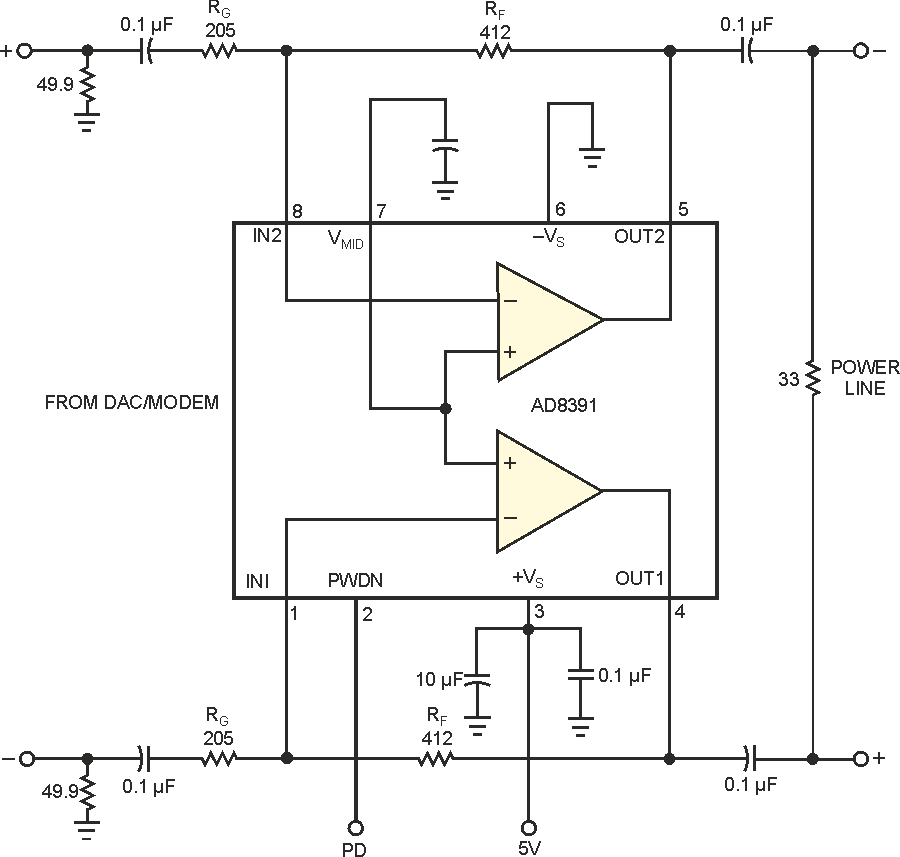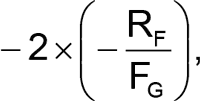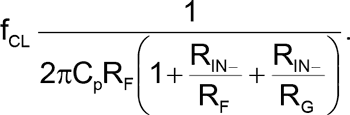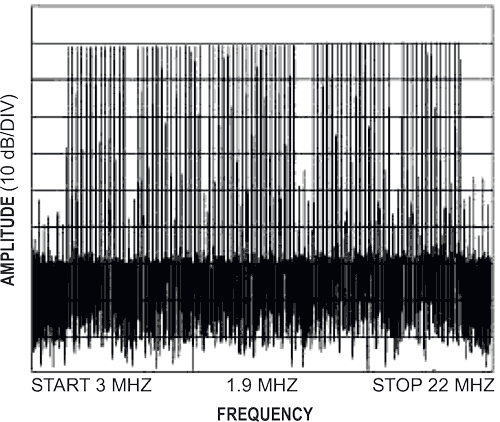# High-current driver serves home-power-line modems

Ryan Metivier, Analog Devices

EDN

Home-based power-line networking signals are similar to xDSL (digitalsubscriber- line) signals in that they both typically employ a form of OFDM (orthogonal frequency-division multiplexing). Both applications require high output current, wide bandwidth, and good linearity. This Design Idea describes a simple line-driver circuit, designed with an xDSL driver, to drive high-speed data over a home power line. Figure 1 shows the AD8391 current-feedback amplifier connected in a negative-feedback circuit to drive wideband, discrete multitone-based signals through home power lines. The advantage of current feedback is that it allows you the flexibility of increasing the gain beyond unity without being limited by the gain-bandwidth product. The AD8391 has 60-MHz bandwidth, 600 V/µsec slew rate and 250-mA output-drive-current capability, making it ideal for driving home power lines.Figure 1. An xDSL driver uses current-feedback technology to make an efficient home-power-line driver.

The circuit in Figure 1 operates with a 5 V supply, has a voltage gain ofand drives a 33 W load. The 33 W load emulates the worst-case impedance of a home power-line network, which can vary greatly from home to home. The driver is transformer-coupled to the power line. The amplitude of the output signal is 2.8 V p-p into the differential power line (hot and neutral) with a peak-to-average ratio of 4 V/V. The feedback resistor, RF, and the gain resistor, RG, maximize circuit bandwidth and stability. For this circuit, an acceptable bandwidth is approximately 30 MHz. The following equation shows the relationship between closed-loop bandwidth (fCL), RG, and RF for current-feedback amplifiers.CP, the internal capacitance, sets the corner frequency of the open-loop transimpedance function, and RIN is the input impedance of the inverting terminal of each amplifier. (Figure 1 does not show CP and RIN.) It is important to note that RF dominates the expression, thus controlling the closed-loop bandwidth. The 49.9 W resistors on the inputs of the circuit terminate the signal source. You should adjust these values based on each application. The four 0.1-µF capacitors provide ac coupling on the input and output lines.Figure 2. The output spectrum of the power-line driver in Figure 1 shows that the worst-case empty-tone distortion is –35 dBc.

The test signal is a composite waveform constructed from the sum of 75 sinusoids of pseudorandom phase. Each tone in the test waveform may have one of four phases to emulate QPSK (quadrature phase-shift keying). The sinusoids are orthogonally spaced from 4 to 21 MHz, leaving the amateur-radio bands empty. Figure 2 shows that the worst-case empty-tone distortion is –35 dBc. This figure is adequate for most practical power-line applications. Figure 3 shows the output in the time domain.Figure 3. This plot represents the time-domain characteristic of the power-line driver.